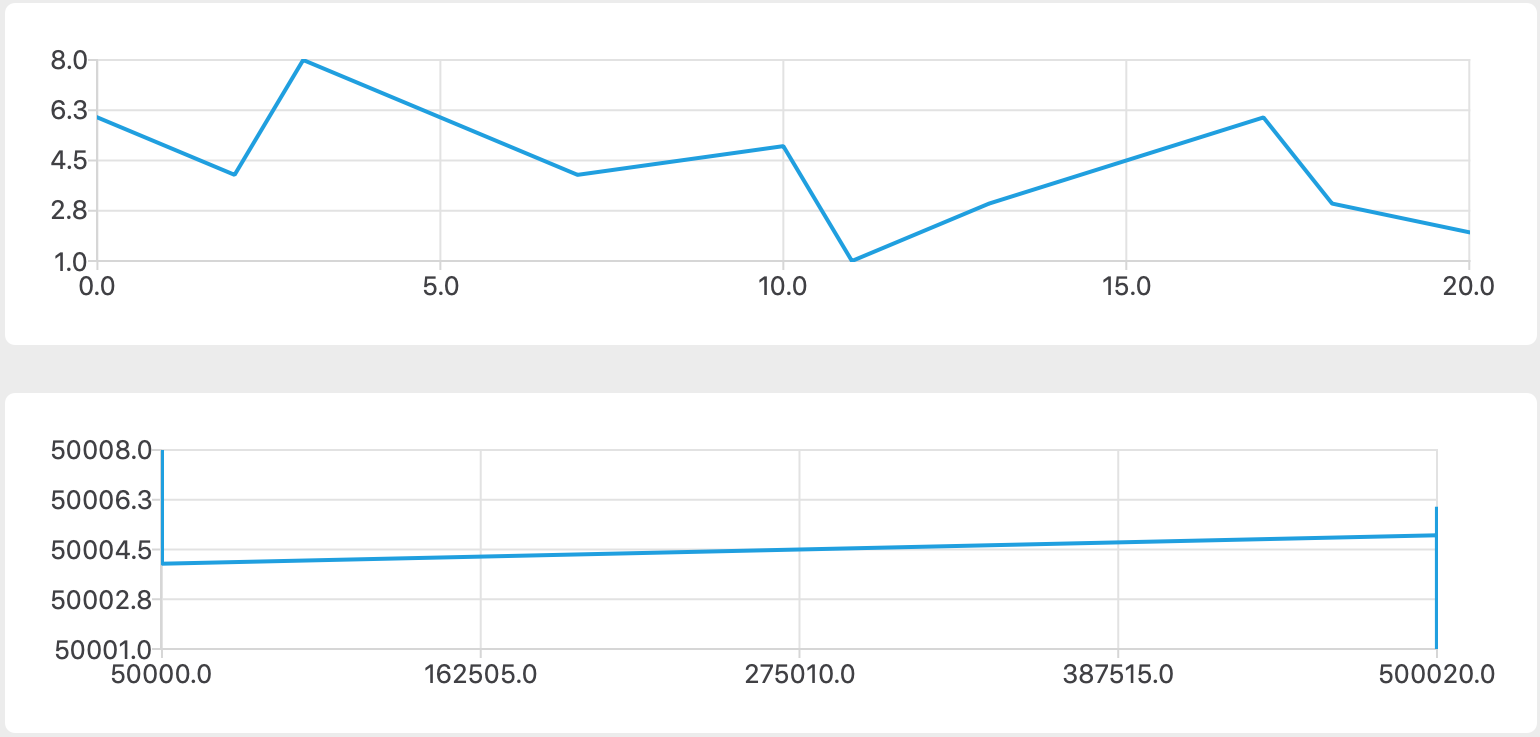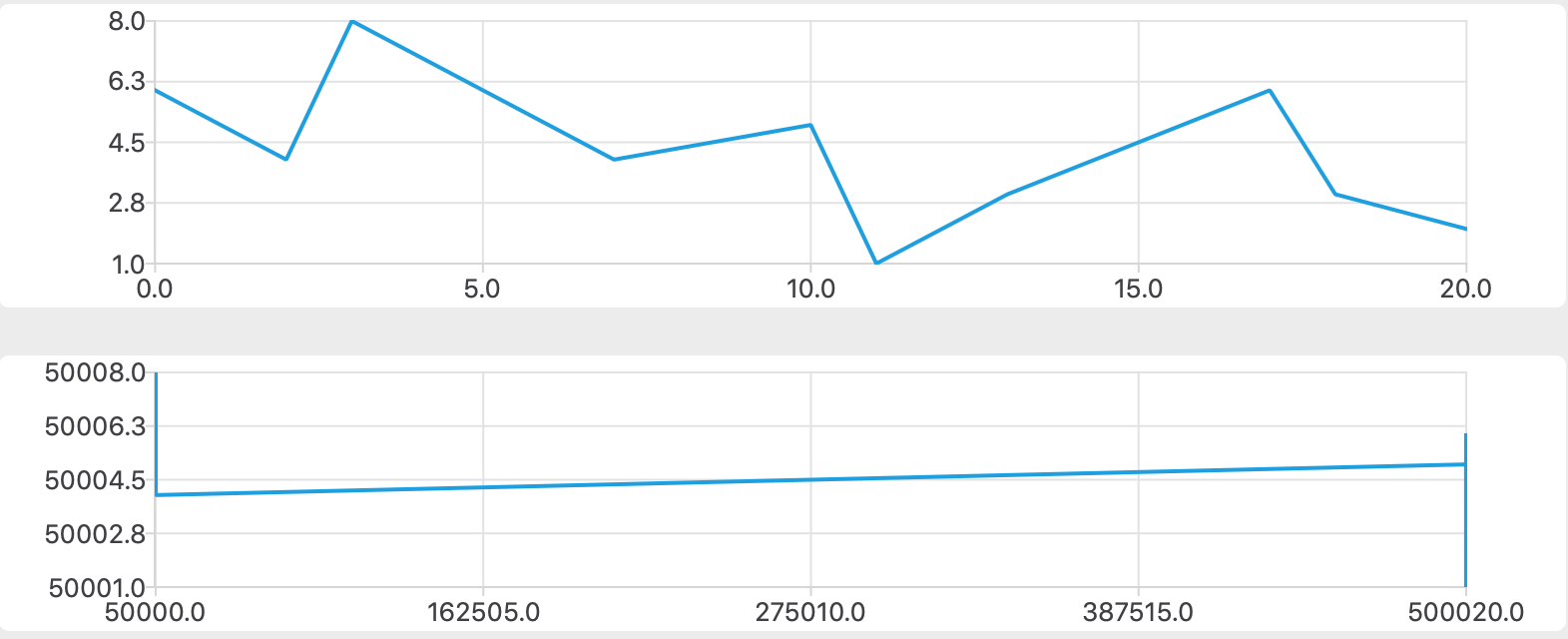Join us on April 18th for a discussion about the top 5 UX/UI Trends shaping the future. Sign-up for the webinar today

# Qt Support - Aligning chart views underneath each other

One thing that comes up occasionally in support is that when you have multiple chart views that it would be good to align them up underneath each other so that the actual plotted size is the same for both charts. If you have similar sizes for the axes then this is easily achieved as they will take up the same width and it will align for you. However, if this is not the case as it so often is, then we can use the margins to force it to have the sizes that we want. So let's say that our chart currently looks like:If you are using C++ for your chart code then this can be achieved with the aid of a single slot, that can be connected to the `QChart::plotAreaChanged()` signal. To start off we need to prevent recursion from happening as we will be changing margins and so on but we want to let the internals still do their thing so we don't want to block signals. So to do that we will just have a static boolean which we will set to true to indicate that we are in the middle of our calculations:

```    void updatePlotArea(const QRectF &area)
{
static bool fixing = false;
if (fixing)
return;
fixing = true;
```

The next thing we need to do is work out what chart is the best one to be used for aligning the others against, this means picking the one that has the largest left value for the plot area (i.e. the one that has the widest axis). We use the currently changed one as a starting point for this and if any are in fact better we change the margins for those as it will ensure we have the most size available for the plot area at any given point for the largest axis.

```    QChart *bestChart = (QChart *)sender();
QRectF bestRect = area;
foreach(QChart *chart, charts) {
if (chart->plotArea().left() > bestRect.left()) {
bestChart = chart;
bestRect = chart->plotArea();
chart->setMargins(QMargins(20, 0, 20, 0));
}
}```

Then, with the exception of the one that ends up being the best one we adjust the margins to ensure that it matches the best one so that they are aligned correctly by setting the margins to be the existing margin plus the difference between the best chart's plot area and this chart's plot area. Finally we send any posted events to ensure it is updated right away for us.

```    foreach(QChart *chart, charts) {
if (bestChart != chart) {
const int left = chart->margins().left() +
(bestRect.left() - chart->plotArea().left());
const int right = chart->margins().right() +
(chart->plotArea().right() - bestRect.right());
chart->setMargins(QMargins(left, 0, right, 0));
}
}
QApplication::sendPostedEvents();
fixing = false;```

This will give us the two charts aligned to look like:As for doing this in QML, we can do something similar in a function which is called via onPlotAreaChanged.

```    property bool fixing: false
property var chartViews: [chartview, chartview_b]
function updatePlotArea(chart, area) {
if (fixing)
return
fixing = true
var tmpChart
var bestRect = chart.plotArea
var bestChart = chart
for (var i = 0; i < chartViews.length; i++) {
tmpChart = chartViews[i]
if (tmpChart.plotArea.left >
Math.ceil(bestRect.left) ||
(Math.ceil(tmpChart.plotArea.left) ===
Math.ceil(bestRect.left) &&
Math.floor(tmpChart.plotArea.right) <
Math.floor(bestRect.right))) {
bestChart = tmpChart;
bestRect = tmpChart.plotArea;
}
}
bestRect.left = Math.ceil(bestRect.left)
bestRect.right = Math.floor(bestRect.right)
for (i = 0; i < chartViews.length; i++) {
tmpChart = chartViews[i]
if (tmpChart !== bestChart) {
var newLeft = 20 + bestRect.left -
Math.floor(tmpChart.plotArea.left);
var newRight = 20 +
Math.ceil(tmpChart.plotArea.right) -
bestRect.right;
tmpChart.margins.left = newLeft
tmpChart.margins.right = newRight
}
}
fixing = false;
}```

The only difference being is that we account for the fact that the plot area is using real values and margins are still integer based so we do some extra accounting for that as a result.

In other news from the support desk, to reiterate the value of reporting bugs directly to the support team as a customer. As a customer indicated the issue reported in https://bugreports.qt.io/browse/QTBUG-74523 we were able to quickly find a solution and also get that integrated in time for the next release. It had been reported earlier this year, but was given a lower priority because Qt Quick Controls 1 is deprecated and therefore no one had scheduled time to investigate it. As always, when a bug is reported to us from a customer it will increase the priority and in cases like this we are able to solve it much quicker as a result as the support team can spend time on it too.